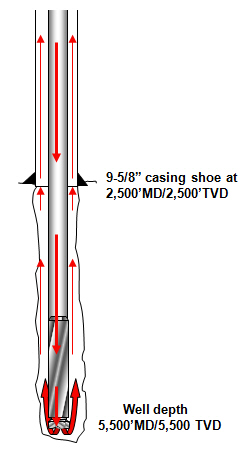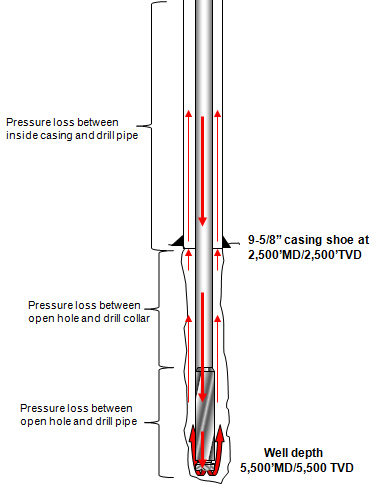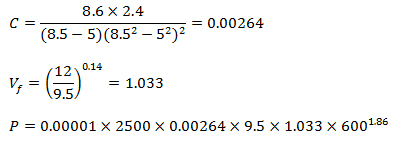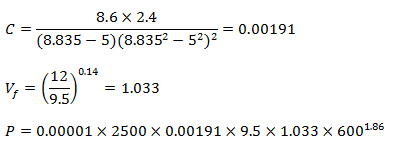# Pressure Losses Through Annulus

Pressure drop in the annulus is another important part of drilling hydraulic calculation and today we would like to demonstrate the equations specifically used to determine pressure loss in the annulus.The following equations are used for calculating pressure loss in the annulus.Assumptions:

Turbulent viscosity = 3 cp

Friction factor = 0.053 based on fully rough passages

Where:

P = pressure loss, psi

L = length of pipe, ft

W = mud density, ppg

Vf = viscosity correction factor

PV = plastic viscosity, centipoises

Q = flow rate, gpm

Dh = hole diameter, inch

Do = Outside diameter of drill pipe or drill collar, inch

C = general coefficient for annulus around drill pipe and drill collar

B = a parameter which takes into account the difference between Q1.86 and Q2. You can just simply select “B” value from the following table.

 Hole Diameter (inch) B Parameter 4-3/4” or less 2.0 5-7/8” – 6-3/4” 2.2 7-3/8” – 7-3/4” 2.3 7-7/8” – 11” 2.4 12” or larger 2.5

Determine the pressure loss in the drill string based on the following information9-5/8” casing shoe at 2500’MD/2500’TVD

ID of 9-5/8” casing = 8.835”

Well depth = 5,500’MD/5,500 TVD

Drill pipe length = 5000 ft

ID of drill pipe = 3.34 inch

OD of drill pipe = 5 inch

Drill collar length = 500 ft

ID of drill collar = 2.8 inch

OD of drill collar = 5 inch

Mud Weight = 9.5 ppg

Plastic Viscosity = 12 centipoise

Flow rate = 600 gpm

Hole size = 8.5 inch

Calculation steps to determine pressure loss in the annulus

Total pressure loss in annulus is composed of three components which are

1) Pressure loss between open hole and drill collar

2) Pressure loss between open hole and drill pipe

3) Pressure loss between inside casing and drill pipePressure loss between open hole and drill collarP = 19 psi

Pressure loss between open hole and drill collar = 19 psi

Pressure loss between open hole and drill pipeP = 95 psi

Pressure loss between open hole and drill pipe = 95 psi

Pressure loss between inside casing and drill pipeP = 69 psi

Pressure loss between inside casing and drill pipe = 69 psi

Total pressure loss in the annulus = 19 + 95 + 69 = 183 psi

Share the joyWorking in the oil field and loving to share knowledge.

### 2 Responses to Pressure Losses Through Annulus

1.Alaa says:

Thanks for the valuable info.

could i know how to compute the required air volume to remove the cuttings from borehole.

Regards

•DrillingFormulas.Com says:

Thanks for your comment but we don’t have any knowledge regarding air drilling.

This site uses Akismet to reduce spam. Learn how your comment data is processed.